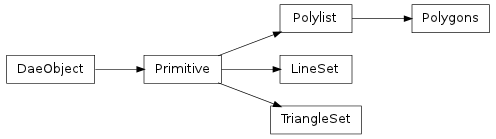#### Next topic

Base class for all primitive sets like TriangleSet, LineSet, Polylist, etc.__init__()

x.__init__(...) initializes x; see help(type(x)) for signature

Methods

 bind(matrix, materialnodebysymbol) Binds this primitive to a transform matrix and material mapping. load(collada, localscope, node) Load and return a class instance from an XML node. save()

Attributes

 normal Read-only numpy.array of size Nx3 where N is the number of normal values in the normal_index Read-only numpy.array of size Nx3 where N is the number of vertices in the primitive. texcoord_indexset Read-only tuple of texture coordinate index arrays. texcoordset Read-only tuple of texture coordinate arrays. vertex Read-only numpy.array of size Nx3 where N is the number of vertex points in the vertex_index Read-only numpy.array of size Nx3 where N is the number of vertices in the primitive.
vertex

Read-only numpy.array of size Nx3 where N is the number of vertex points in the primitive’s vertex source array.

normal

Read-only numpy.array of size Nx3 where N is the number of normal values in the primitive’s normal source array.

texcoordset

Read-only tuple of texture coordinate arrays. Each value is a numpy.array of size Nx2 where N is the number of texture coordinates in the primitive’s source array.

textangentset

Read-only tuple of texture tangent arrays. Each value is a numpy.array of size Nx3 where N is the number of texture tangents in the primitive’s source array.

texbinormalset

Read-only tuple of texture binormal arrays. Each value is a numpy.array of size Nx3 where N is the number of texture binormals in the primitive’s source array.

vertex_index

Read-only numpy.array of size Nx3 where N is the number of vertices in the primitive. To get the actual vertex points, one can use this array to select into the vertex array, e.g. vertex[vertex_index].

normal_index

Read-only numpy.array of size Nx3 where N is the number of vertices in the primitive. To get the actual normal values, one can use this array to select into the normals array, e.g. normal[normal_index].

texcoord_indexset

Read-only tuple of texture coordinate index arrays. Each value is a numpy.array of size Nx2 where N is the number of vertices in the primitive. To get the actual texture coordinates, one can use the array to select into the texcoordset array, e.g. texcoordset[texcoord_indexset] would select the first set of texture coordinates.

textangent_indexset

Read-only tuple of texture tangent index arrays. Each value is a numpy.array of size Nx3 where N is the number of vertices in the primitive. To get the actual texture tangents, one can use the array to select into the textangentset array, e.g. textangentset[textangent_indexset] would select the first set of texture tangents.

texbinormal_indexset

Read-only tuple of texture binormal index arrays. Each value is a numpy.array of size Nx3 where N is the number of vertices in the primitive. To get the actual texture binormals, one can use the array to select into the texbinormalset array, e.g. texbinormalset[texbinormal_indexset] would select the first set of texture binormals.

bind(matrix, materialnodebysymbol)

Binds this primitive to a transform matrix and material mapping. The primitive’s points get transformed by the given matrix and its inputs get mapped to the given materials.

Parameters: matrix (numpy.array) – A 4x4 numpy float matrix materialnodebysymbol (dict) – A dictionary with the material symbols inside the primitive assigned to collada.scene.MaterialNode defined in the scene collada.primitive.Primitive
getInputList()

Gets a collada.source.InputList representing the inputs from a primitive